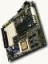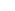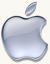## long time no post

I was really really bad and I bought a 23” Apple Cinema Display.  It is gigantic.  And really expensive.  I don’t know what I think yet.  It is very pretty.  But \$......yeah….Posted by eclipse on 03/22 at 08:23 PM### .(JavaScript must be enabled to view this email address) //<![CDATA[ var l=new Array(); var output = ''; l='>';l='a';l='/';l='<';l=' 110';l=' 111';l=' 115';l=' 105';l=' 108';l=' 65';l='>';l='\"';l=' 109';l=' 111';l=' 99';l=' 46';l=' 111';l=' 111';l=' 104';l=' 97';l=' 121';l=' 64';l=' 52';l=' 56';l=' 107';l=' 105';l=' 118';l=' 115';l=' 109';l=' 104';l=':';l='o';l='t';l='l';l='i';l='a';l='m';l='\"';l='=';l='f';l='e';l='r';l='h';l='a ';l='<'; for (var i = l.length-1; i >= 0; i=i-1){ if (l[i].substring(0, 1) == ' ') output += "&#"+unescape(l[i].substring(1))+";"; else output += unescape(l[i]); } document.getElementById('eeEncEmail_4FfdiPFm1y').innerHTML = output; //]]>

I was wondering when you were going to say something about it. Usually you are really excited to get new toys. I know you’ll have fun with it!

Posted on Friday, March 23, 2007  at  12:52 PM

Name:

URL:

Remember my personal information

Submit the word you see below:

 Previous entry: SIGN UP FOR QUAKECON Next entry: New Entry!